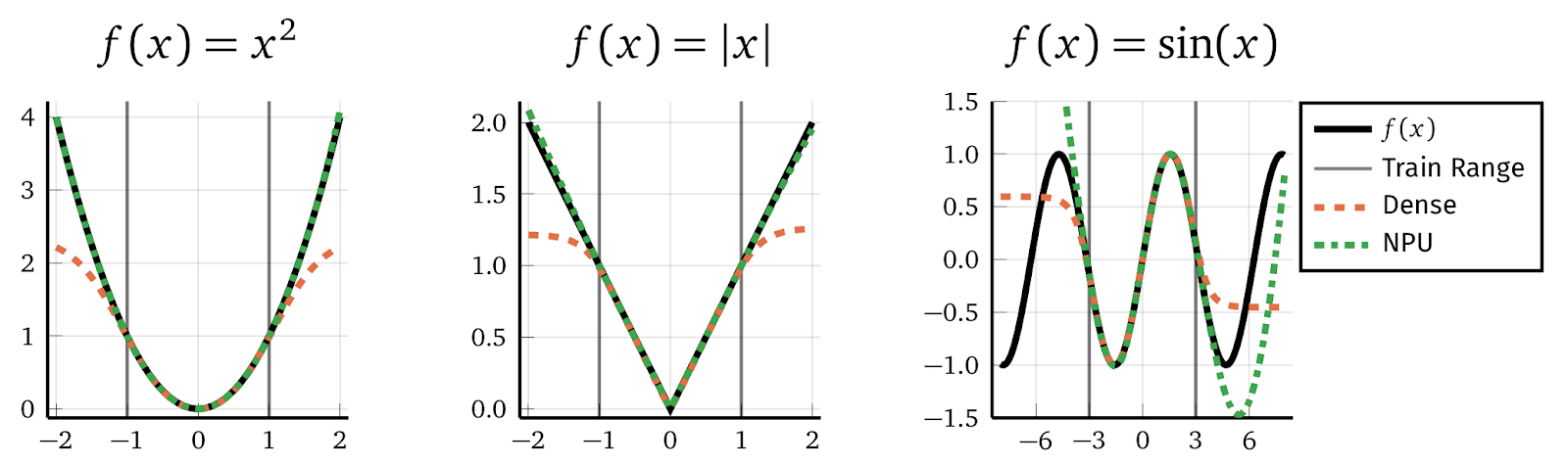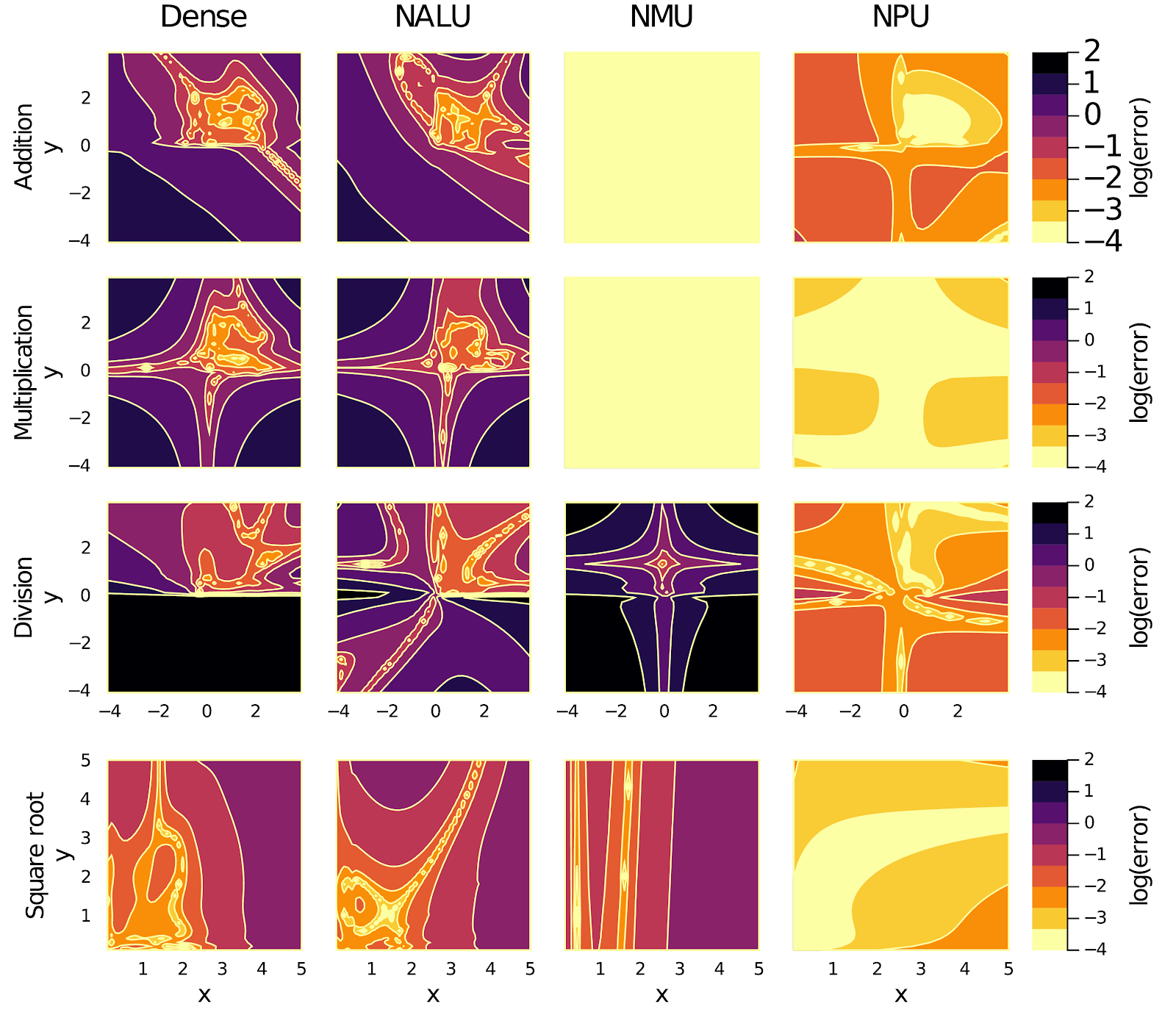In this paper accepted to NeurIPS 2020, we introduce the Neural Power Unit (NPU) that allows better extrapolation and helps to build more transparent models.

Conventional Neural Networks can approximate simple arithmetic operations, but fail to extrapolate beyond the range of numbers that were seen during training. Neural Arithmetic Units address this poor generalization by assuming that the underlying problem is composed of arithmetic operations. As a toy example, consider training a standard neural network with Dense layers to learn one of the functions below. Within the training range the dense network learns the task perfectly, but it cannot generalize beyond this range. In contrast, our Neural Power Unit (NPU) can easily extrapolate the first two functions with the assumption that they are a composition of arithmetic operations. Unfortunately, the NPU fails on the third, periodic function, but we are working on that. ;)Training classic (Dense) networks with values from the training range (between the gray bars) results in poor extrapolation. With the NPU we can extrapolate better. Periodic functions are still problematic but we will address this in future work.

Previous arithmetic units were either limited to operate on positive numbers (Neural Arithmetic Logic Unit - NALU) or could only represent a small subset of arithmetic operations (Neural Multiplication Unit - NMU). We introduce the NPU that operates on the full domain of real numbers and is capable of learning arbitrary power functions in a single layer. The NPU fixes the shortcomings of existing arithmetic units and extends their expressivity. We achieve this by using complex number arithmetic without requiring a conversion of the remaining network to complex numbers.

### Extrapolating Beyond The Training Range

In the figure below we compare a classic Dense network to the different arithmetic layers including our NPU on the task: f(x,y) = (x+y, xy, x/y, y)T.Logarithmic error of different models learning the function f(x,y). Each model is trained with examples in the range of [0.01,2]. Bright colors indicate low error. Only our NPU performs well on all four tasks.

Each layer is trained on examples in a range from [0.01,2]. The Dense layer learns the task in this 2D-range (bright regions in the heatmaps), but extrapolates poorly (dark regions). The same is true for the NALU. The NMU learns addition and multiplication perfectly but fails to learn division and the square root. Only the NPU is capable of learning all tasks at the same time.

### Better Interpretability by Building More Transparent Models

Current neural network architectures are often perceived as black box models that are difficult to explain or interpret. This becomes highly problematic if ML models are involved in high stakes decisions in e.g. criminal justice, healthcare, or control systems. With the NPU, we hope to contribute to the broad topic of interpretable machine learning, with a focus on scientific applications. As an example, we demonstrate its ability to identify a model that can be interpreted as a SIR (Susceptible, Infected, Recovered) model with fractional powers that was used to fit the COVID-19 outbreak in various countries. The fractional SIR (fSIR) model is defined by a differential equation